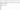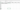# Delete Excel Rows using PowerShell

## Problem#

In this blog post, we will show you how to delete Excel rows using PowerShell.

As the context, our original Worksheet will contain data as follows:And we will delete all the rows except the first one which is a column header as follows:## Using Excel Com Object#

Since PowerShell is deeply integrated with .NET Framework, we can use `ComObject` to work with Excel files.

After creating the object, we will open the Excel file and get the range used within a worksheet. This range will contain the rows in which we will loop through backwardly because when we delete a row the next row will be shifted above. We only delete the rows other than the first one.

``````
try {
# Open excel file
\$excel = New-Object -ComObject Excel.Application
\$workbook = \$excel.Workbooks.Open("C:\Scripts\Test.xlsx")

# Get all the rows within used range in a worksheet
\$rows = \$workbook.Worksheets.Item(1).UsedRange.Rows

# Loop backwardly through all the rows and Delete them except the first one which is a column header
for (\$i = \$rows.Count; \$i -gt 0; \$i--) {
if (\$rows[\$i].Row -ne 1) {
\$rows[\$i].Delete()
}
}

# Save excel file
\$workbook.Save()
}
finally {
# Close excel file
\$workbook.Close()

\$excel.Quit()
[System.Runtime.Interopservices.Marshal]::ReleaseComObject(\$excel)
}

``````

We enclose the code above with `try-finally` block because we want to make sure it will release the resource after modifying Excel that is performed at `finally` block.

## Using ImportExcel Module#

`ImportExcel` is an external module that is built based on EPPlus which is a very well-known library to work with Excel spreadsheets in .NET.

Before using `ImportExcel` module, we have to install it.

``````
Install-Module -Name ImportExcel

``````

And then following previous solution pattern, we have to create Excel object first before removing the rows. We will perform deletion using `DeleteRow` method of workSheet object.

``````
try {
# Import the module
Import-Module ImportExcel

# Open excel file
\$excel = Open-ExcelPackage -Path "C:\Scripts\Test.xlsx"

# Get all the rows within used range in a worksheet
\$workSheet = \$excel.Workbook.Worksheets
\$startRow = \$workSheet.Dimension.Start.Row
\$endRow = \$workSheet.Dimension.End.Row

# Loop backwardly through all the rows and Delete them except the first one which is a column header
for (\$i = \$endRow; \$i -ge \$startRow; \$i--) {
if (\$i -ne 1) {
\$workSheet.DeleteRow(\$i)
}
}
}
finally {
# Close excel file
Close-ExcelPackage \$excel
}

``````

Similar to previous solution, we enclose the script with `try-finally` block to avoid memory leak.

## Using PSExcel Module#

`PSExcel` is another module based on EPPlus library. You can find all the examples in github repository.

Before using this module, we have to install it.

``````
Install-Module -Name PSExcel

``````

In order to use the object, we must import the module first. Then, we create Excel object by specifying the path of our Excel file. This object will be used to get the range being used in a worksheet. This range contains the information about the first and the last row.

Based on this, we will loop through all the rows in backward manner to delete the rows except the first one.

``````
try {
# Import the module
Import-Module PSExcel

# Open excel file
\$excel = New-Excel -Path 'C:\Scripts\Test.xlsx'

# Get all the rows within used range in a worksheet
\$workSheet = \$excel.Workbook.Worksheets
\$startRow = \$workSheet.Dimension.Start.Row
\$endRow = \$workSheet.Dimension.End.Row

# Loop backwardly through all the rows and Delete them except the first one which is a column header
for (\$i = \$endRow; \$i -ge \$startRow; \$i--) {
if (\$i -ne 1) {
\$workSheet.DeleteRow(\$i)
}
}

# Save excel file
\$excel | Save-Excel
}
finally {
\$excel | Close-Excel
}

``````

We also enclose the script with `try-finally` block to avoid memory leak.

## Conclusion#

To delete Excel rows using PowerShell, we can use `Excel Com Object` which is based on .NET Framework. We can also use PowerShell external modules like `PSExcel` and `ImportExcel`. `ImportExcel` is an excellent module which is based on `EPPlus` library, a well-known C# libary for woking with Excel from .NET.

`PSExcel` is the alternative of `ImportExcel` module. `PSExcel` is also based on `EPPlus` but this module is no longer maintained as stated in its GitHub repository. If it doesn’t provide functionality that you need, you should contribute to the source code by yourself.